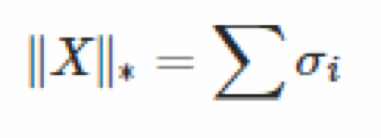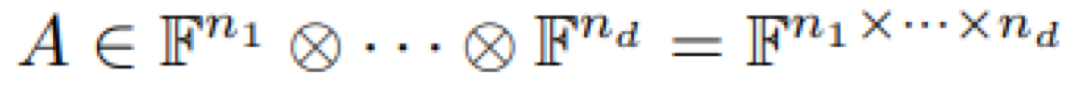# Tensor Nuclear Norms

What is Tensor Nuclear Norm:

A tensor’s nuclear norm is the sum of its singular values, as provided by the singular value decomposition (SVD) of the tensor itself. This is important in dimensionality reduction and multi-variate statistics, where the nuclear norm of a 2-tensor is used as convex surrogate for dimensional rank, which dramatically simplifies many deep learning optimization problems.

How is a Tensor Nuclear Norm Calculated?

The nuclear norm of a 2-tensor x * x (a matrix) can be defined as:Where σi is the ii-th singular value of X.

The nuclear norm of a d-tensor, when F = R or C is:It’s calculated by solving for:where ∥ · ∥ is the l2-norm and uk,i ∈ Fnk for k = 1,...,d, i = 1,...,r.

The nuclear norm is found when d = 2, which is equivalent to the standard definition as a sum of singular values.